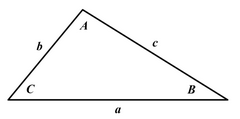## Solutions of Triangle Calculator

Variable Input Solution Solution 2
Angle in degrees.
Area (square units):
Status:

You need input 3 pieces of information.

Three sides (abbreviated SSS)

Two sides and the included angle (abbreviated SAS)

A side and the two angles adjacent to it (abbreviated ASA)

Two sides and an angle not included between them (abbreviated SSA)

A side, an angle adjacent to it and the angle opposite to it (abbreviated AAS).For example, To Side side angle (SSA) case, side a = 18, side b =24, Angle A =45°, The solution one: Side c = 22.9705627, Angle B = 70.5287794°, Angle C = 64.4712206°, Area = 194.911688. The solution two: Side c = 10.9705627, Angle B = 109.471221°, Angle C = 64.4712206°, 25.5287794°, Area = 93.0883118.

For example, To Side side angle (SSA) case, side a = 13, side b = 12, Angle B = 30, The solution one: Side c = 21.3454508, Angle A = 32.7971683°, Angle C = 117.202832°, Area = 69.3727149. The solution two: Side c = 1.17120975, Angle A = 147.202832°, Angle C = 2.79716830°, Area = 3.80643168.

Thinkcalculator.com provides you helpful and handy calculator resources.